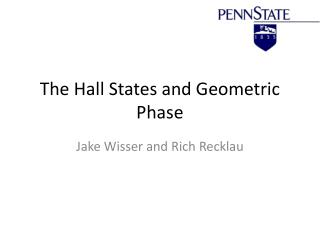# The Hall States and Geometric Phase - PowerPoint PPT PresentationDownload PresentationThe Hall States and Geometric Phase

The Hall States and Geometric PhaseDownload Presentation## The Hall States and Geometric Phase

- - - - - - - - - - - - - - - - - - - - - - - - - - - E N D - - - - - - - - - - - - - - - - - - - - - - - - - - -
##### Presentation Transcript

1. The Hall States and Geometric Phase Jake Wisser and Rich Recklau

2. Outline • Ordinary and Anomalous Hall Effects • The Aharonov-Bohm Effect and Berry Phase • Topological Insulators and the Quantum Hall Trio • The Quantum Anomalous Hall Effect • Future Directions

3. I. The Ordinary and Anomalous Hall Effects Hall, E. H., 1879, Amer. J. Math. 2, 287

4. The Ordinary Hall Effect VH Charged particles moving through a magnetic field experience a force Force causes a build up of charge on the sides of the material, and a potential across it

5. The Anomalous Hall Effect VH “Pressing effect” much greater in ferromagnetic materials Additional term predicts Hall voltage in the absence of a magnetic field

6. Anomalous Hall Data Where ρxx is the longitudinal resistivity and β is 1 or 2

7. II. The Aharonov-Bohm Effect and Berry Phase Curvature

8. Vector Potentials Maxwell’s Equations can also be written in terms of vector potentials A and φ

9. Schrödinger’s Equation for an Electron travelling around a Solenoid Where For a solenoid Solution: Where ψ’ solves the Schrodinger’s equation in the absence of a vector potential Key: A wave function in the presence of a vector potential picks up an additional phase relating to the integral around the potential

10. Vector Potentials and Interference If no magnetic field, phase difference is equal to the difference in path length If we turn on the magnetic field: There is an additional phase difference!

11. Experimental Realization Interference fringes due to biprism Critical condition: Due to magnetic flux tapering in the whisker, we expect to see a tilt in the fringes Useful to measure extremely small magnetic fluxes

12. Berry Phase Curvature For electrons in a periodic lattice potential: The vector potential in k-space is: Berry Curvature (Ω) defined as: Phase difference of an electron moving in a closed path in k-space: An electron moving in a potential with non-zero Berry curvature picks up a phase!

13. A Classical Analog Non-Zero Berry Curvature Zero Berry Curvature Parallel transport of a vector on a curved surface ending at the starting point results in a phase shift!

14. Anomalous Velocity VH E Systems with a non-zero Berry Curvature acquire a velocity component perpendicular to the electric field! How do we get a non-zero Berry Curvature? By breaking time reversal symmetry

15. Time Reversal Symmetry (TRS) Time reversal (τ) reverses the arrow of time A system is said to have time reversal symmetry if nothing changes when time is reversed Even quantities with respect to TRS: Odd quantities with respect to TRS:

16. III. The Quantum Trio and Topological Insulators

17. The Quantum Hall Trio

18. The Quantum Hall Effect • Nobel Prize Klaus von Klitzing (1985) • At low T and large B • Hall Voltage vs. Magnetic Field nonlinear • The RH=VH/I is quantized • RH=Rk/n • Rk=h/e2 =25,813 ohms, n=1,2,3,…

19. What changes in the Quantum Hall Effect? • Radius r= m*v/qB • Increasing B, decreases r • As collisions increase, Hall resistance increases • Pauli Exclusion Principle • Orbital radii are quantized (by de Broglie wavelengths)

20. The Quantum Spin Hall Effect

21. The Quantum Spin Hall Effect König et, al

22. What is a Topological Insulator (TI)? Bi2Se3 Insulating bulk, conducting surface

23. V. The Quantum Anomalous Hall Effect

24. Breaking TRS • Breaking TRS suppresses one of the channels in the spin Hall state • Addition of magnetic moment • Cr(Bi1-xSbx)2Te3

25. Observations No magnetic field! As resistance in the lateral direction becomes quantized, longitudinal resistance goes to zero Vg0 corresponds to a Fermi level in the gap and a new topological state

26. VI. Future Directions

27. References • http://journals.aps.org/pr/pdf/10.1103/PhysRev.115.485 • http://phy.ntnu.edu.tw/~changmc/Paper/wp.pdf • http://mafija.fmf.uni-lj.si/seminar/files/2010_2011/seminar_aharonov.pdf • https://www.princeton.edu/~npo/Publications/publicatn_08-10/09AnomalousHallEffect_RMP.pdf • http://physics.gu.se/~tfkhj/Durstberger.pdf • http://journals.aps.org/prl/abstract/10.1103/PhysRevLett.5.3 • http://journals.aps.org/rmp/abstract/10.1103/RevModPhys.25.151 • http://www-personal.umich.edu/~sunkai/teaching/Fall_2012/chapter3_part8.pdf • https://www.sciencemag.org/content/318/5851/758 • https://www.sciencemag.org/content/340/6129/167 • http://www.sciencemag.org/content/318/5851/766.abstract • http://www.physics.upenn.edu/~kane/pubs/p69.pdf • http://www.nature.com/nature/journal/v464/n7286/full/nature08916.html • http://www.sciencemag.org/content/340/6129/153